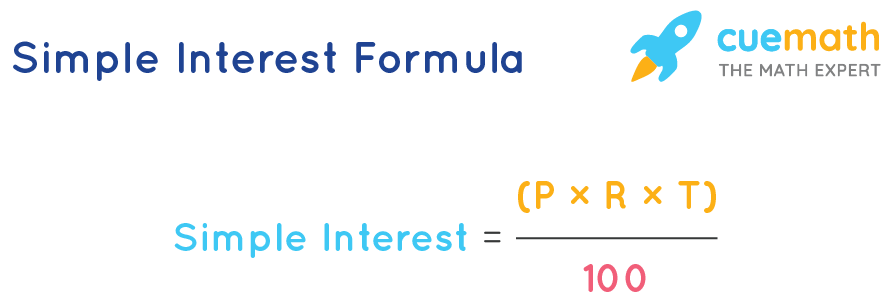# Simple Interest Formula

Using the simple interest formula, one can calculate the percentage of the principal amount, charged by the lender or bank to the borrower for the use of its assets or money for a specific time period. In this section, we will be discussing the various aspects of the simple interest formula, and understand the variables involved.

## What is the Simple Interest Formula?

The simple interest for a given amount can be calculated by the following formulas,

Simple Interest = (P*R*T)/100where,

P = Principal amount

R = Rate of interest

T = Time period

Let's take a quick look at a couple of examples to understand the simple interest formula, better.

## Solved Examples Using Simple Interest Formula

### Example 1:

What is the simple interest on the principal amount of $12000 in 2 years, if the interest rate is 12%? Solution: To find: Simple Interest after 2 years. Principle amount =$12000, r = 12%, t = 2(given)

Using the simple interest formula,

SI = (P*R*T)/100

SI = (12000*12*2)/100

SI = 2880

Answer: Simple interest after the end of 2 years will be $2880. ### Example 2: James borrowed$600 from the bank at some rate per annum and that amount becomes double in 2 years. Calculate the rate at which James borrowed the money.

Solution:

To find: Interest rate

Principle amount = $600, Amount =$1200, Time = 2 year(given)

Using the simple interest formula,

Simple interest = Amount - Principal

Simple interest = $1200-$600= \$600

Again using the simple interest formula,

SI = (P*R*T)/100

600 = (600*R*2)/100

R = 50

Answer: James borrowed the money at 50% rate.